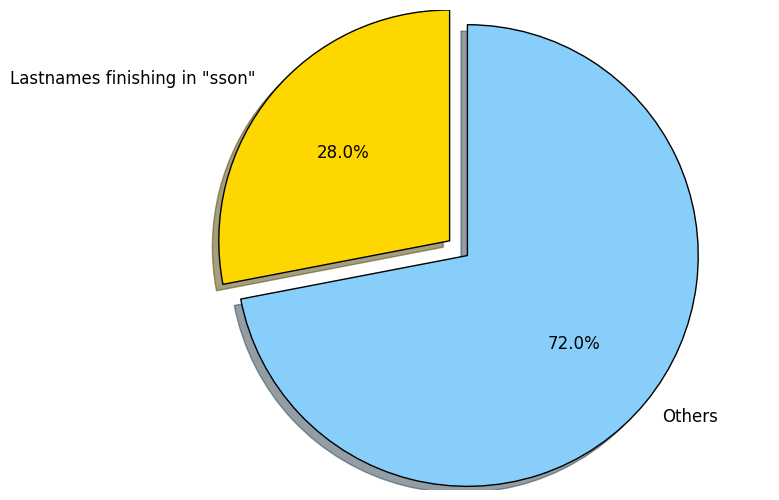# MalmöMilen 2014 post analysis¶

Since I took part in the 2014 edition of MalmöMilen (a 10km race in Malmö, Sweden), one of the things I have wanted to do for a long time was to step back and have a look at the results. There are accessible from this page, which is easy to scrap and analyze.

Let's do that step by step.

## Toolbox¶

Let's group all the tools at the same place, so the next cells focus on the logic.

In :
import requests
from bs4 import BeautifulSoup
from collections import Counter

import math
import matplotlib.pyplot as plt;
from matplotlib.ticker import FuncFormatter
% matplotlib inline
plt.rcdefaults()

import numpy as np

import itertools
import statistics as stats
import datetime


## Data scraping¶

The data source is divided into 12 different web pages for men, 9 for women, so the web page needs to be scraped several times. Let's define a function for that.

In :
raceNumber = {"M": 8364, "W": 8541}

def scrap_page(gender, runners, p):
page = requests.get("http://www.racetimer.se/en/race/resultlist/1997?checkpoint=9999&layout=marathon&page=" + str(p) + "&rc_id=" + str(raceNumber[gender]))
soup = BeautifulSoup(page.text)
if (soup == None):
return
table = soup.body.table.find(id='top3-list')
lines = table.find_all('tr')

if len(lines) == 0:
return

for line in lines:
c = line.find_all('td')
if len(c) == 0:
continue

r = {}
r["rank"] = int(c.text)
r["fullname"] = c.text.replace("»", "").strip()
split = r["fullname"].rfind(" ")
r["firstname"] = r["fullname"][:split].strip()
r["lastname"] = r["fullname"][split+1:].strip()
r["city"] = c.text.strip()

# get a proper value for the year of birth
try:
r["yob"] = int(c.text.strip()) if c.text.strip() != "" else -1
except ValueError:
r["yob"] = -1

r["nb"] = int(c.text.strip())
r["time"] = c.text.strip()

# convert the time to seconds
parts = r["time"].split(':')
hours, minutes, seconds = 0, 0, 0
if len(parts) == 2:
minutes, seconds = parts
else:
hours, minutes, seconds = parts
r["timeInSeconds"] = int(hours) * 3600 + int(minutes) * 60 + int(seconds)

runners[gender].append(r)


## Number of runners¶

Let's run that to fetch all the runners, men and women

In :
runners = { "M": [], "W": [] }

for i in range(1, 13):
scrap_page("M", runners, i)
scrap_page("W", runners, i)

total_men = len(runners["M"])
total_women = len(runners["W"])
print("Men:", total_men)
print("Women:", total_women)

Men: 2982
Women: 2150


## Time range¶

In :
for g in runners.keys():
print(g, ": first in " + runners[g]["time"] + " , last in " + runners[g][-1]["time"])

W : first in 35:59 , last in 1:40:46
M : first in 29:54 , last in 1:43:10


List the most popular firstnames for men and women, with the number of occurences

In :
top = 10
men = Counter([p["firstname"] for p in runners["M"]])
top_men = men.most_common(top)
for i, m in enumerate(top_men):
print(str(i+1) + ". " + m + ": " + str(m))

1. Johan: 101
2. Anders: 83
3. Fredrik: 82
4. Peter: 72
5. Martin: 69
6. Mikael: 68
7. Magnus: 64
8. Daniel: 64
9. Jonas: 57
10. Andreas: 54

In :
women = Counter([p["firstname"] for p in runners["W"]])
top_women = women.most_common(top)
for i, w in enumerate(top_women):
print(str(i+1) + ". " + w + ": " + str(w))

1. Anna: 90
2. Maria: 62
3. Jenny: 43
4. Malin: 40
5. Karin: 37
6. Emma: 36
7. Sara: 35
8. Cecilia: 32
9. Annika: 32
10. Johanna: 32


## Time distribution¶

One interesting stat is to compare how the runners expected to perform to how they actually performed.

Let's redraw the previous start group distribution, which corresponds to the runners own pronostic. The following graph displays the time the participants thought it will take for them to complete the race.

### Start group distribution¶

In :
url = 'http://www.malmomilen.se/anmaelan/startlista-2014'
page = requests.get(url)
soup = BeautifulSoup(page.text)
table = soup.body.table.find_all('tr')

ps = {}
for line in table:
c = line.find_all('td')
time = c.text if c.text != '' else 'N/A'
if time not in ps:
ps[time] = 0
ps[time] += 1

sorted_groups = ['Elitgruppen', 'Under 42 minuter', 'Under 45 minuter', '45-50 minuter',
'50-55 minuter', '55-60 minuter', '60+ minuter', 'Barnloppet']
groups = [g[:8] for g in sorted_groups]
y_pos = np.arange(len(groups))[::-1]
n = [ps[group] for group in groups]

rects = plt.barh(y_pos, n, align='center', alpha=0.5)
plt.yticks(y_pos, sorted_groups)
plt.xlabel('Number of participants')
plt.title('Start group distribution')

for i, rect in enumerate(rects):
plt.text(0.95 * rect.get_width(), rect.get_y() + rect.get_height() / 2.0, ps[groups[i]], ha='right', va='center')

plt.show()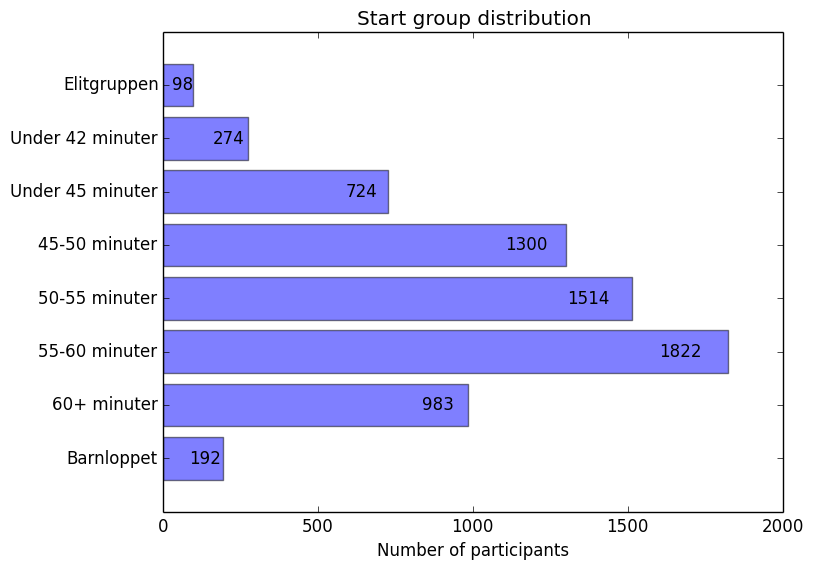### Post race time distribution¶

In comparison, we display the same graph, but this time with the final values. Note that there might be a small gap between the number of registered runners and the number of people who actually finished the race.

But anyway the graph still gives a pretty good idea of how the race went, and some differences are quite noticeable.

For example the "elite runners" seem to be quite modest, while a few hundreds of runners more did it in more than 60 minutes.

In :
all_runners = runners["M"] + runners["W"]
intervals = [(0, 40), (41, 42), (43, 45), (46, 50), (51, 55), (56, 60), (61, 200)]
ids =  * 1000
for i, inter in enumerate(intervals):
ids[inter:inter] = [i] * (inter - inter + 1)

c = Counter([ids[int(math.ceil(r["timeInSeconds"] / 60))] for r in all_runners])
results = [cnt for cnt in c.items()]

# remove barnloppet for this analysis
final_groups = sorted_groups[:-1]
final_y_pos = np.arange(len(final_groups))[::-1]

rects = plt.barh(final_y_pos, results, align='center', alpha=0.6)
plt.yticks(final_y_pos, final_groups)
plt.xlabel('Number of participants')
plt.title('Final time distribution')

for i, rect in enumerate(rects):
plt.text(0.95 * rect.get_width(), rect.get_y() + rect.get_height() / 2.0, results[i], ha='right', va='center')
plt.show()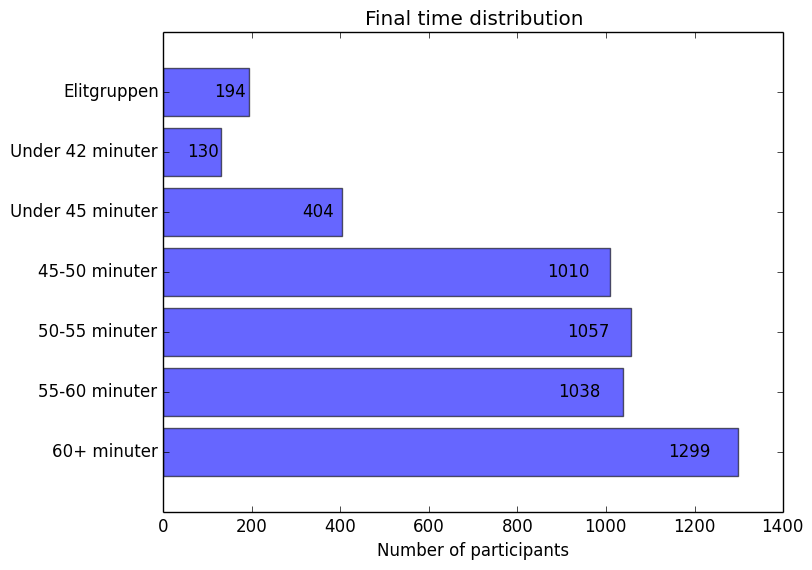## Has the year of birth an influence on the time?¶

The data is not 100% good, some year of birth are missing. Let's remove them.

In :
# remove runners without a proper year of birth
weird_yob = [r for r in all_runners if r["yob"] == -1 or r["yob"] >= 2014 or r["yob"] < 1914]
all_runners_yob = [r for r in all_runners if r not in weird_yob]

print(str(len(weird_yob)) + " people have a weird year of birth ... Examples: " + str([r["yob"] for r in weird_yob[:15]]))

256 people have a weird year of birth ... Examples: [2203, 6711, 6703, -1, -1, -1, -1, -1, -1, -1, -1, 7307, -1, 6008, 9010]

In :
group_by_yob = itertools.groupby(sorted(all_runners_yob, key=lambda x: x["yob"]), lambda x: x["yob"])
avg_by_yob = [(k, stats.mean([r["timeInSeconds"] for r in g])) for k, g in group_by_yob]

def secondsToHumanTime(x, pos):
return str(datetime.timedelta(seconds=x))

avg_by_yob = sorted(avg_by_yob, key=lambda x: x)

yob_x = [x for x in avg_by_yob]
yob_y = [x for x in avg_by_yob]

plt.xlabel('Year of birth')
plt.ylabel('Average time per year of birth')
plt.title('Variation of the time given the year of birth')

plt.plot(yob_x, yob_y, 'bo')
plt.gca().yaxis.set_major_formatter(FuncFormatter(secondsToHumanTime))
plt.show()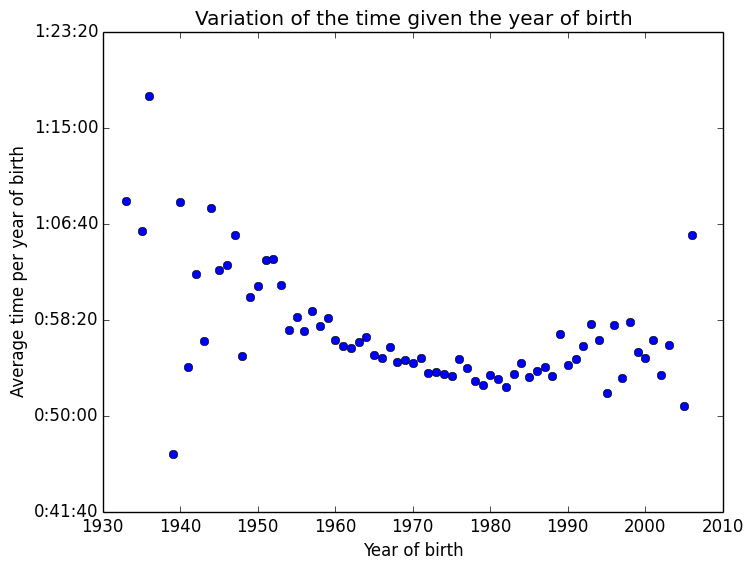The time value plotted above represents the average time per year. The age distribution is maybe weird, let's have a look at it.

In :
group_by_yob = itertools.groupby(sorted(all_runners_yob, key=lambda x: x["yob"]), lambda x: x["yob"])
sum_by_yob = [(k, len(list(g))) for k, g in group_by_yob]

sum_x = [x for x in sum_by_yob]
sum_y = [x for x in sum_by_yob]

plt.xlabel('Year of birth')
plt.ylabel('Number of runners')
plt.title('Number of runners per year of birth')

plt.plot(sum_x, sum_y, 'bo', linewidth=2)
plt.show()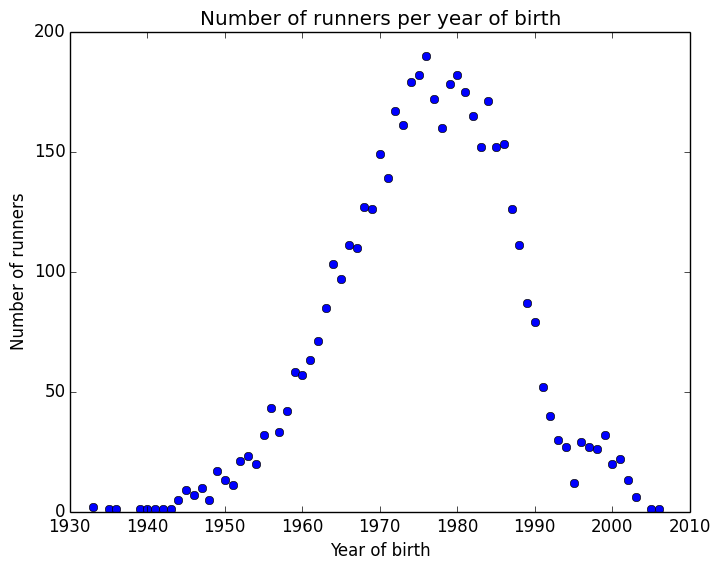## Fun facts¶

### How many people with a lastname finishing by "sson"?¶

In :
total_number = len(all_runners)
total_sson = sum([1 if r["lastname"][-4:] == "sson" else 0 for r in all_runners])
print(str(total_sson) + " runners have a lastname finishing with \"sson\". Welcome to Sweden.")

labels = 'Lastnames finishing in "sson"', 'Others'
sizes = [total_sson, total_number - total_sson]
colors = ['gold', 'lightskyblue']
explode = (0.1, 0)

plt.pie(sizes, explode=explode, labels=labels, colors=colors, autopct='%1.1f%%', shadow=True, startangle=90)
plt.axis('equal')

plt.show()

1438 runners have a lastname finishing with "sson". Welcome to Sweden.